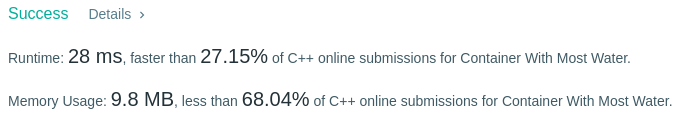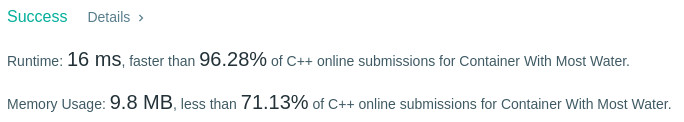# Coding Spirit

0%

Algorithm Puzzles everyday every week sometimes: Container With Most Water

## Puzzle

Puzzle from leetcode:

Given n non-negative integers a1, a2, …, an , where each represents a point at coordinate (i, ai). n vertical lines are drawn such that the two endpoints of line i is at (i, ai) and (i, 0). Find two lines, which together with x-axis forms a container, such that the container contains the most water.

Note: You may not slant the container and n is at least 2.

## Solution

This puzzle can be easily solved by exhaustion method but time complexity is O(N^2). A better approach will be using “compress window“：

Here time complexity is O(N)Try to use iterator:Bravo!